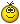# Mohanaji's rangoli on paper

Tried Mohanaji's pattern on the paper with colored pencils.

I've used one more level other than Mohanaji. Here is the level of complexity:

n = 0, no of lines = 0, no of dots = 1
n = 1, no of lines = 1 (1 green), no of dots = 5
n = 2, no of lines = 5 (4 green, 1 pink), no of dots = 25
n = 3, no of lines = 9 (4 green, 4 pink, 1 blue), no of dots = 61
n = 4, no of lines = 13 (4 green, 4 pink, 4 blue, 1 purple), no of dots = 113
n = 5, no of lines=17, (4 green, 4 pink, 4 blue, 4 purple, 1 orange) no of dots = 181

Thank you for the workout, Mohanaji.Hi diya....simply superbkeep it up.....Wow diya wonderful creation dear...I already write the following calculations to JKM sir's rangoli. Just want to share with u dear...To my little brainI analyzed and found some calculations. I can not express it in mathematical words. So I write them in my style. Pardon me if any mistakes are there...n = 0, lines 0, dot 1
n = 1, lines 1 ( 0+1), dots 5 ( 1+ 4), difference 5- 1= 4 ( 0+ 4)
n = 2, lines 5 ( 1+4), dots 25 ( 5+ 20), difference 25- 5= 20 ( 4+16)
n = 3, lines 9 ( 5+4), dots 61 ( 25+ 36), difference 61- 25= 36 ( 20+16)
n = 4, lines 13 ( 9+4), dots 113 ( 61+ 52), difference 113- 61= 52 ( 36+16)
n = 5, lines 17 (13+4), dots 181 (113+ 68), difference 181-113= 68 ( 52+16)
n = 6, lines 21 (17+4), dots 265 (181+ 84), difference 265-181= 84 ( 68+16)
n = 7, lines 25 (21+4), dots 365 (265+100), difference 365-265=100 ( 84+16)
n = 8, lines 29 (25+4), dots 481 (365+116), difference 481-365=116 (100+16)
n = 9, lines 33 (29+4), dots 613 (481+132), difference 613-481=132 (116+16)
n =10, lines 37 (33+4), dots 761 (613+148), difference 761-613=148 (132+16)

The line count may be achieved from adding the previous level lines with 4.
The dots count may be achieved from adding the previous level of dots with the difference between the two level of dots added with number 16.

I think, We can go indefinitely with n value. I can not found the relationship of n with the line and dot counts ...Can u please explain it...Wonderful diyA! I am glad that young people like you are interested in this art. Drawing on the graph paper really helps. Regards! - mOhanaSuperb diya dearnice and neat try on graph...well done kolam diya.Superb Diya......pretty one diya,,, nicelooks pretty....thank you sooooo much Diya(for the help) :bigsmile:Wow..Admiring your work so much Diya. Well done wtih nice colour combination and done with so much neatness and perfection. Carry on your work !!! :star: :star: :star: